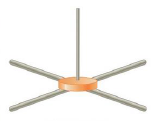Chapter 8, Problem 41P

Chapter
Section
Textbook Problem

An approximate model for a ceiling fan consists of a cylindrical disk with four thin rods extending from the disk’s center, as in Figure P8.41. The disk has mass 2.50 kg and radius 0.200 m. Each rod has mass 0.850 kg and is 0.750 m long, (a) Find the ceiling fan’s moment of inertia about a vertical axis through the disk’s center, (b) Friction exerts a constant torque of magnitude 0.115 N · m on the fan as it rotates. Find the magnitude of the constant torque provided by the fan’s motor if the fan starts from rest and takes 15.0 s and 18.5 full revolutions to reach its maximum speed.Figure P8.41

(a)

To determine
The ceiling fan’s moment of inertia about vertical axis through the disk’s center.

Explanation
The moment of inertia of the ceiling fan is defined as I=Idisk+4Irod and further it can be written as I=(1/2)MdRd2+4[(MrLr2)/3] .

Given info: The mass of the disk is 2.50kg , radius of the disk is 0.200m , mass of each rod is 0.850kg , and the length of these rods is 0.750m .

The formula for the ceiling fan’s moment of inertia about vertical axis through the disk’s center is,

I=MdRd22+43MrLr2

• Md is mass of the disk.
• Rd is radius of the disk.
• Mr is mass of the rod.
• Lr is length of the rod

(b)

To determine
The magnitude of the constant torque provided by the fan’s motor.

Still sussing out bartleby?

Check out a sample textbook solution.

See a sample solution

The Solution to Your Study Problems

Bartleby provides explanations to thousands of textbook problems written by our experts, many with advanced degrees!

Get Started